# Analysis of the Impact of Effective Length of Morning Glory Spillway on Its Performance (Numerical Study)

Analysis of the Impact of Effective Length of Morning Glory Spillway on Its Performance (Numerical Study)
Faculty of Civil Engineering, Semnan University, Semnan, Iran

Faculty of Civil Engineering, University of Sabzevar, Razavi Khorasan Province, Iran

Corresponding Author Email:
hkarami@semnan.ac.ir
Page:
211-221
|
DOI:
https://doi.org/10.18280/i2m.180217
12 December 2018
|
Accepted:
5 April 2019
|
Published:
30 June 2019
| Citation

OPEN ACCESS

Abstract:

The Morning Glory spillway is one of the types of spillways that are used for the passage of excess waters and floods from the dams' upstream to the downstream of the dams. Numerical simulations and examination have been rarely done on the sectoral Morning Glory spillways, which are used due to special conditions in the topography of the construction site. In this research, the simulation of the flow through the sectoral morning glory spillway was done using the three-dimensional numerical model. The characteristics of flow in this type of spillways were investigated and a relation was proposed for the rate of water discharge. To do this, five sector angles were considered in the geometry of the spillway crest and the characteristics of the flow, as well as the amount of drainage capacity in them were compared. Finally, a relation was provided to calculate the discharge flow rate of the sectoral morning glory spillways. The results obtained from the relation indicated that the maximum error of the calculations with the help of the relation provided is equal to 7.77 % and occurs at a sector of 62 degrees. This error percentage confirms the reliability of the relation.

Keywords:

computational fluids dynamics (CFD), finite volume method, sectoral morning glory spillways, spillway discharge capacity, 3D modeling

1. Introduction

One of the crises that countries with low rainfall rate and abundant drought, including Iran, are now faced with provides water for drinking, agriculture, industry, and other uses.

One of the ways to collect surface waters is through the use of water structures, including dams. Due to the limited availability of groundwater resources and running out of the aquifers, the importance of such structures becomes more obvious.

Reservoir dams are one of the most important structures that are established to store surface waters. Due to the high volume of water stored in these dams, their proper design as well as the design of related structures, such as spillways, which are responsible for the passage of destructive flows from the dam reservoir, seems to be of great importance.

Each dam has many lateral structures, by operation of which together, the highest dam efficiency rate can be obtained.

The flood drainage structure is one of the most important structures of the dam. These structures can include lower outlets drains and spillways, which are responsible for the discharge of excess water resulting from floods.

Spillways and bottom outlets need to be designed to discharge a volume of water equal to the largest possible flood in the catchment area of the dam in a proper period time. If they do not operate well, the excess water passes over the dam crest and will cause significant damages to the dam and its subsidiary facilities. In some cases, it may well destroy the whole dam.

The morning glory spillway is one of the few structures separate from the dam body, which consists of a funnel-like opening and a vertical and horizontal shaft. It is used in places where other types of spillway cannot be built.

The design of this kind of spillway is done in a specific way since the hydraulic behavior of this structure is related to the topography of the surroundings, diameter, and geometry of the crown, the downstream curve or arch and the dimensions of the lower discharge tunnel.

As mentioned, the crest geometry of this type of spillway influences the flood discharge capacity. Thus, the turbulence and eddy currents while passing the crest can be decreased by making changes in the crest geometry, and subsequently, increase the discharge capacity of the overflow.

Morning glory spillways are usually used in embankment dams. Due to a high number of embankment dams, a significant number of these types of spillways have been used in dams.

So far, some research has been done on the improvement of flood flow capacity in morning glory spillways, including those such as creating steps in the spillway crest, changing the shape of the spillway crest to the triangular, pentagon, and seven-faceted funnels, some of which were referred to in the study.

Fattor and Bacchiega (2009) conducted some investigations on the Potrerillos dam in Argentina. They were able to determine a better location for locating the morning glory spillway to provide more suitable hydraulic conditions compared with the spillway built in this dam. Also, in their studies, they proposed better boundary conditions for the surroundings of the morning glory spillway. In this study, some evaluations were made on vortex breaker blades and their required number to improve the spillway drainage capacity of this dam. Their proposed model also produced favorable hydraulic conditions with a significant reduction in the required excavations around the spillway .

Savic et al. (2014) at the Belgrade University of Serbia examined the discharge flow rate by embedding a deflector at the downstream of the morning glory spillway crest in several dams in Serbia with the help of a laboratory prototype made from the original model. They finally presented an algorithm for designing the morning glory spillways containing deflector .

Nohani (2015) performed a study by using a laboratory physical model to determine the flow rate coefficient passing through the morning glory spillways under geometric conditions of the edge of the spillway crest (sharp-crested and broad-crested). In this study, the morning glory spillways with different diameters and with and without vortices breakage elements at the spillway crown were used and investigated .

By building a parabolic knee joint not previously used and placing it at the end of the vertical shaft in the morning glory spillway, Fais et al. (2015) studied the hydraulic behavior of this type of knee joint compared to the 90-degree knee joint and multi-center knee joint. The results showed that the parabolic knee joint made by them increases the discharge capacity of the morning glory spillway .

Xianqi (2015) focused on studying the flow profile in the morning glory spillways of large dams. In this study, the geometric and hydraulic characteristics of several large dams with morning glory spillways were selected and the maximum outflow rates were extracted on each one as a case study. Then, using a physical model, five different geometric and hydraulic designs were applied .

Alfatlawi and Alshakli (2015) focused on the prediction of coefficient of discharge for stepped morning glory spillway. For this purpose, two spillway's shapes studied experimentally, namely, circular and quadrate. Each shape stepped into 4, 5 and 6 steps experimental models. Experimental results used later to predict the coefficient of discharge by the benefit of Artificial Neural Network (ANN) and Multiple Nonlinear Regression (MNLR) approaches. Results show that coefficient of discharge of stepped morning glory spillway decreased with the increasing (head/length) and/or (head/radius) ratios .

Nohani (2015) by using a numerical model, investigated the hydraulic parameters of the flow such as coefficient of discharge, water level profile and pressure distribution and compared with the data obtained from USACE and USBR models. As the result, he found that, although comparing the parameters shows a good and show agreement between the results, however, using the numerical model in real samples has limitations such as the number and size of the network, computational time and accurate modeling of the weather .

Shemshi and Kabiri (2016) investigated swirling flow at vertical shaft spillways with an innovative geometry, circular piano-key (CPK) inlet, based on model experimentation. The results showed that the swirling flow strength for flow through the CPK spillway is several times lower than that for simple shaft spillways .

Musavi-Jahromi et al. (2016) conducted 170 experiments using different numbers and the angle of vortex breakers which are established on the physical model of this spillway in KWP in order to determine their effect on the increment of its discharge coefficient rate. The results of the tests showed that establishing six vortex breakers with the angle of 45 ̊ on the spillway is the most efficient arrangement for this aspect .

In some cases, due to existing constraints at the site of the construction of the spillway or because of increasing the drainage capacity from the weirs, the spillway crest geometry is seen to undergo changes and transforms from the conventional funnel form into other forms.

In sectoral spillways, as their name suggests, a sector of the full crest circle is used to discharge the water. Such overflows are used for various reasons such as the lack of sufficient space for constructing a full morning glory spillway or no need to build a full-diameter morning glory spillway and the sufficiency of a sector of the overflow for passing the probable floods (Figure 1).

## 1-.png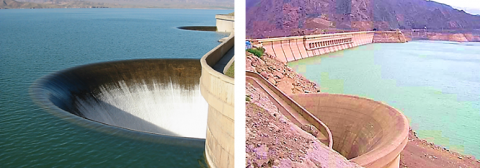Figure 1. An example of a sectoral morning glory spillway constructed in the Manjil dam (Sefid Rud River Dam) in Iran [10-11]

A review of previous studies indicates that most studies have been done on non-sectoral morning glory spillways and improving the discharge conditions. However, there seems to be no research on the radial (sectoral) morning glory spillways. In this paper, the characteristics of flow in the radial (sectoral) morning glory spillways were studied and a relation was proposed to determine the discharge rate of the sectoral morning glory spillway.

2. Materials & Methods

An available laboratory model, as well as the numerical model, was used in this research, which is described in the following.

2.1 Laboratory model used

The available laboratory results were used to verify the numerical model. It should be noted that the data available on the full morning glory spillway were used due to the lack of laboratory results related to the radial (sectoral) morning glory spillway.

## 2-.pngFigure 2. A view of the model built from the morning glory spillway of Haraz dam before and after impounding 

Haraz Dam is one of the dams under construction in Iran, in which, the morning glory spillways are used to passing flood to downstream. Haraz Dam is located at 20 km from the south of city of Amol on the Haraz River and the goals of its construction include providing agricultural water and power generation. The laboratory model of the morning glory spillway of this dam was made in the Laboratory of the Water Research Institute of the Ministry of Energy. The hydraulic behavior of this structure has been examined in the reports of this laboratory (Figure 2).

In laboratory modeling, the studied sample was made with a scale of 1:30. According to the scale mentioned, the levels of the lake water surface for flood discharge rate passing over the spillway in the model were measured at a distance of 4 meters (120 meters in the actual sample) from the axis of the spillway crest in the dam reservoir lake . The details and specifications of the dam and the morning glory spillway of Haraz Dam are presented in Table 1.

Table 1. Details and specifications of the dam and the morning glory spillway of Haraz dam 

 Characteristic Dimension Characteristic Dimension Height (from base) 150 m Spillway inlet diameter 33.76 m Dam crest length 377 m Spillway vertical shaft diameter 8.3 m Height from the bed 120 m Height from the crest edge to the spillway vertical shaft 26.92 m Dam body volume 6.10 million cubic meters Height from the beginning of the vertical shaft to the beginning of the knee joint 48.17 m Reservoir volume at normal water level 240 million cubic meters Knee joint height 18.74 m Useful (in service) volume 151 million cubic meters Length of the horizontal tunnel (horizontal shaft) 544.05 m Spillway horizontal tunnel slope 0.0389 Vertical diameter of the horizontal tunnel 7.5 m

The results extracted from the experimental model included discharge capacity (stage-discharge) where the water of the lake was recorded at 502.51 to 507.40 levels at 22 different altitude levels and the flow rates (discharges) corresponding to these altitude values were recorded (Figure 3).

## 3.pngFigure 3. The results of discharge capacity (stage-discharge) of the morning glory spillway of Haraz Dam 

3. Numerical Model

The numerical simulation of the morning glory spillway of Haraz Dam was performed based on the reference laboratory model . The geometry of the model, including the crest, vertical shaft, conversion (turning) section and knee joint, the primary section of the horizontal tunnel, the aeration tube as well as the topography of the area adjacent to the morning glory spillway were recalled to the Flow Analyzer Software (FLOW-3D) after drawing for hydraulic analysis.

3.1 Model meshing

For this simulation, the conditions of viscose and incompressible fluid, the entry of air with a density of 1.2 kg/m3 and the shear stress coefficient of 0.073 were considered. The mesh blocks were used to mesh the model in the FLOW-3D software where the dimensions of the mesh cells can be chosen in them. In this model, a large mesh block was used for the reservoir section. The smaller mesh blocks were used in the section of the vertical shaft, transform section, the knee joint, and the airing tube for a more accurate solution as shown in Figures 4 and 5.

## 4.png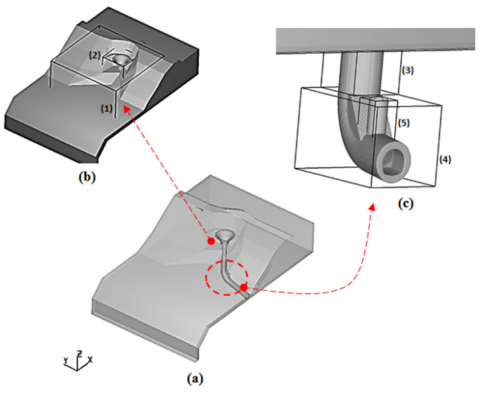Figure 4. The formation of the mesh blocks in the model

## 5.pngFigure 5. A view of the size of the mesh cells in the mesh block No. 2

3.2 Boundary conditions

The boundary conditions in the mesh block 1 were applied to the hydraulic model according to the coordinate axis indicated in Figure 4 in the Y Max and Y Min directions in the experimental model with the impermeable wall. Therefore, in this numerical model, the boundary conditions of the wall were chosen for the walls, which are quite similar to the real conditions.

The boundary conditions of the Z min floor were considered symmetrical in the mesh block. The reason for considering these boundary conditions for this side of the mesh block is that in the FLOW-3D software, due to the FAVOR feature, the rigid sections of the model geometry are detected. Thus, there is no need to consider the boundary conditions of Wall for this side. To apply boundary conditions for the Z Max mode in the numerical model, given the fact that in the actual model, the flow has a free surface in direct contact with the air, the symmetrical boundary conditions must be used for this mode. In this case, the gradient of changes in the air pressure is considered zero inside and outside.

Since in the FLOW-3D model, we do not need to define the air phase as a new phase in hydraulic problems, the free surface flow conditions and direct contact with the relative pressure of the air are quite established. To correctly select and apply the input and output boundary conditions in the X Min and X Max sections, steady flow with a specific height from the fluid over the spillways has to be introduced into a numerical model according to real studies. Therefore, using the boundary conditions in the FLOW-3D numerical model, the fluid height for the X Min mode with the Fluid Height boundary conditions is applied to this section, which exactly conforms to the laboratory model, using which, the results are verified.

On the other hand, after the flow passes over the spillway, the flow must be directed to the outlet boundary and outside the numerical model. The outlet flow in the downstream was applied as Outflow as in the case of the hydraulic model conditions. It should be noted that all of the above boundary conditions were applied to the larger block that encompasses the entire computational space. The internal blocks and the conversion and knee joint part have symmetrical boundary conditions to prevent any effect on the model in terms of boundary conditions.

4. Governing Equations and the Computational Scheme

The equations of RANS (Reynolds-averaged Navier–Stokes) and continuity used generally in case of incompressible flow, involving VOF and FAVOR variables are described in Eq. (1) and (2):

• Continuity:

$\frac{\partial}{\partial x}\left(u A_{x}\right)+\frac{\partial}{\partial y}\left(v A_{y}\right)+\frac{\partial}{\partial z}\left(w A_{z}\right)=0$    (1)

• Momentum:

$\frac{\partial U_{i}}{\partial t}+\frac{1}{V_{F}}\left(U_{j} A_{j} \frac{\partial U_{i}}{d x_{j}}\right)=\frac{1}{\rho} \frac{\partial P^{\prime}}{d x_{i}}+g_{i}+f_{i}$    (2)

where, variables u, v, and w are velocities in the x-, y-, and z-directions, VF is fluid volume fraction in each cell, Ax, Ay and Az are fractional areas that the flow can pass through in the mentioned directions, ρ is Density, ρ' is Pressure, gi is the gravitational force in the mentioned directions, fi is Reynolds stresses which needs a turbulence model to create the closure.

As seen, the VF and Aj (cell face areas) are equivalent to one in cells filled completely with fluid. Hence, the equations are reduced to the basic incompressible RANS equations.

5. Model Preparation for Flow Analysis

In the studied numerical model, increasing the flow analysis time in the software settings made the flow approaching an almost steady mode after 140 seconds; but, the analysis time in model calibration was set to 200 seconds to reduce the error and lower the fluctuations in the flow rate.

5.1 Turbulence model

A comparison was done between LES, k-ε, and RNG turbulence models to select the appropriate turbulence model for simulation. In this comparison, the reservoir water level was considered in the elevation of 503.86 m (Figure 3) to compare the distortion rate of the results in each of these disturbance models with the reported amounts in the laboratory as shown in Figure 6.

## 6.png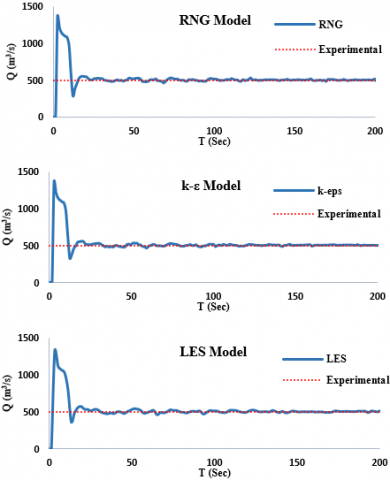Figure 6. Comparison of RNG, k-eps and LES models in calculating the discharge flow rate of the morning glory spillway

As shown in Figure 6, the results of the software analysis are almost the same for the turbulence models and there is no significant difference in the results. Given the similar results in these three models of turbulence, the RNG model was selected for calibrating the model since the calculations made by the software ended in this turbulence model in a period time about 20 % less than two other turbulence models.

5.2 Mesh sensitivity measurement

Mesh sensitivity measurement in the model was conducted using the stage-discharge results provided by the Laboratory of the Water Research Institute of the Ministry of Energy , in which the outlet flow rates from the morning glory spillway have been reported in 22 different water elevation of the dam reservoir. To this end, the water level of 503.86 m was considered (Figure 3) and the sensitivity is measured by resizing the mesh cells. For measuring the mesh sensitivity, the dimensions of the mesh cells were initially chosen equal to 1.15 m with a number of 365666 cells (as the largest dimension of mesh cells). Then, the size of the cells was lowered. These dimensions were considered as primary dimensions as size larger than these values for mesh cells did not cover the small thicknesses like the walls of the vertical shafts and the geometry of the model in the FAVOR section was not identified by the software. Then, respectively, dimensions of 1 meter (428501 cells), 0.92 m (478462 cells), 0.85 m (538491 cells), 0.75 m (674784 cells) and 0.65 m (892457 cells) were considered for mesh cells. The simulation results for these sizes are shown in Figure 7.

## 7-33.png## 7-2.png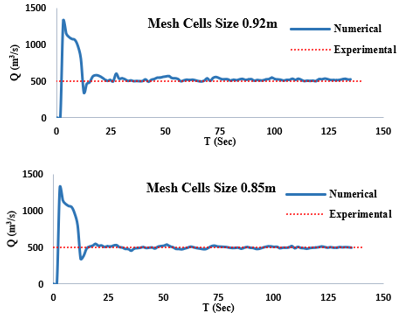## 7-3.png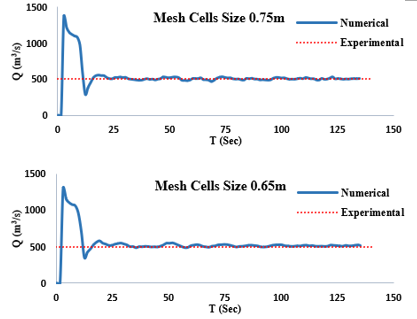Figure 7. Evaluation of the mesh sensitivity by shrinking mesh cell dimensions

By shrinking the size of the mesh cells (Figure 7), it is known that the discharge flow rate of the morning glory spillway is modified and tends to match with the value reported by the lab. The discharge rate in the cell's dimensions of 1.15 m. has a very high error and moves toward the laboratory value by lowering the mesh size up to 0.75 meters and remains intact in smaller amounts than that and lowering the cells dimensions will not affect the discharge flow rate anymore. Therefore, given the ineffectiveness of lowering mesh dimensions and increased model analysis time in smaller cells size, the 0.75 meters size was used in the calibration of the model for mesh cells. The mesh blocks of this model with this mesh size will include 674784 cells.

5.3 Calibrating the model

To calibrate the model with respect to the elevation of reservoir water considered in the laboratory, five water elevation were considered for the numerical model, which were analyzed and are shown in Table 2. The results of the numerical analysis of the model at these five levels in comparison with the quantities extracted in the laboratory are given in Figure 8.

## 8-1.png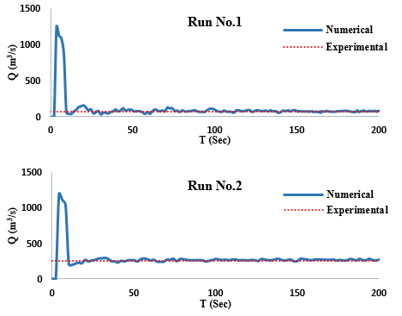## 8-2.png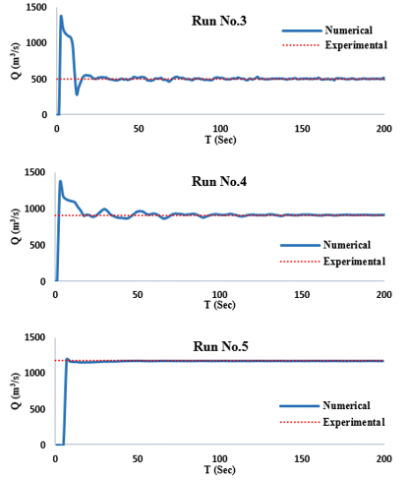Figure 8. Flow (discharge) rate changes based on time in five selected water elevation in the software and their comparison with laboratory values

Table 2. Hydraulic properties of the numerical model simulated from the morning glory of Haraz Dam

 Run No. Elv (m) h (m) Q Exp (m3/s) Q Num (m3/s) Err (%) 1 502.51 0.51 70.5 77.1 -9.36 2 503.2 1.2 253.25 263 -3.85 3 503.86 1.86 501.22 489.8 2.28 4 504.76 2.76 908.46 924 -1.71 5 505.48 3.48 1172.37 1139.85 2.77

The values of the flow height over the spillway crest and the discharge flow rates in the numerical and laboratory models are provided in Table 2. In this table, Elv is the elevation of the free surface of the fluid and h the spillway crest distance from the free flow surface, while QExp and QNum are the flow (discharge) rates extracted from the experimental and numerical models, respectively. The error rate (% Err) was also calculated from Eq. (3).

Error%=100× $\frac {Q_{E x p} - Q_{N u m}} {Q_{E x p}}$                    (3)

According to Table 2 and Figure 9, one can conclude that the present numerical model has calculated the outflow discharge values from the spillway with a maximum error of 9.36 % and the discharge correlation obtained from the laboratory and the numerical model (R2=0.9987) is almost linear. It should be noted that the maximum simulation error occurs in lower discharge rates as the flow, in general, is not formed on the bed of the ovoid profile as in the real model.

The percentage of errors obtained from comparing the output discharge rates taken from the software and lab suggest that the simulation is reliable and the numerical model is calibrated. Using the results obtained in the validation stage, the radial (sectoral) morning glory spillway was then modeled.

## 9.pngFigure 9. Correlation analysis (R2) of the flow rates obtained from the software and the laboratory

6. Modeling of Radial (Sectoral) Morning Glory Spillway

To draw the radial (sectoral) morning glory spillway, the complete radial (sectoral) morning glory spillway was initially considered from the plan view (without a sector) as shown in Figure 10. Then, to select the sections of the morning glory spillway, first, the hypotenuse ab was drawn a tangent to the horizontal section of the vertical shaft to determine the largest sector on the crest of spillway overflow (choosing the larger hypotenuses blocks the vertical shaft, which is not the subject of this study). Drawing the ab hypotenuse, the aôb angle is formed. Then, to draw three other sectors, a line segment was drawn from the middle of the ab hypotenuse perpendicular to the spillway circumference, which cut the spillway crest circumference at the point i. This line segment was divided into four equal parts and the hypotenuses of cd, ef and gh were drawn parallel to the hypotenuse ab at these points to form angles of côd, eôf and gôh (point o is the center of the circle).

## 10.png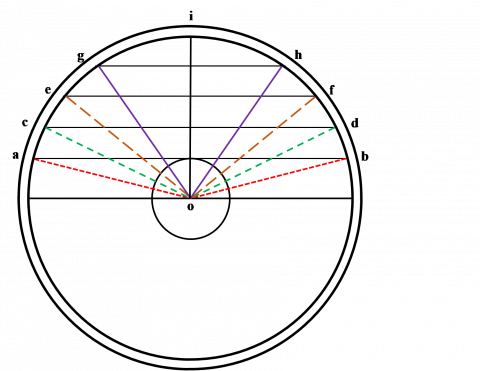Figure 10. A view of the morning glory spillway crest plan and how to choose the crest sectors

The angles of the sectors considered for the morning glory spillway geometry are given in Table 3.

Table 3. The values of selected angles for the drawing of the morning glory spillway sectors

 Row Angle name (θ) Value (Degree) 1 aôb 152 2 côd 127 3 eôf 99 4 gôh 62

According to the selected angles shown in Table 3, the geometry of the sectoral morning glory spillways was drawn and transmitted to the FLOW-3D software for hydraulic analysis. In Figure 11, the geometry of the sectoral morning glory spillways is shown.

## 11-.pngFigure 11. A view of the geometry of the sectoral morning glory spillways modeled in this study

As shown in Figure 11, five angles were selected to investigate the sectoral morning glory spillways in this research. In these five geometries formed, after impounding at three different water elevations in the dam reservoir, the effects of changing the geometry of spillway on its hydraulic behavior were investigated.

These three elevations for the dam reservoir were selected in such a way to cover three modes of flow by the crest control (area (1)), tube or orifice control (area (2)) and pipe control (area (3)). To this end, three water elevations were considered with the mentioned conditions as described in Table 4. Figure 12 also shows a view of the spillway with water.

Table 4. Selected altitude levels for the numerical model

 Discharge controlling conditions Altitude values of the lake water (m) Control at crest 503.86 Tube or orifice control 505.09 Pipe control 505.48

## 12xin_.png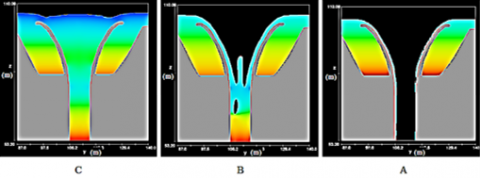Figure 12. Side view of the morning glory spillway; (A) By applying the elevation levels of 503.86 m for the water of the lake (control at the crest); (B) by applying a height value of 505.09 meters for the lake water (Tube or orifice control); (C) by applying a height value of 505.48 meters for the lake water (Pipe control)

7. Results Analysis

In this section, some hydraulic characteristics of sectorial (radial) morning glory spillway, including spillway discharge capacity and velocity vector, are investigated, and an equation for calculating the discharge rate is presented.

7.1 Flow characteristics analysis in the morning glory spillway, considering sectors

7.1.1 Calculating discharge capacity of the morning glory spillway

The three-dimensional sectoral models made with three height levels (Table 4) in the FLOW-3D software were impounded and analyzed. The discharge rate flow results for these spillways are shown in Table 5.

Where, θ is the angle of the sector, Le is effective length of the sectoral spillway, Elv is elevation of the fluid free surface, h is distance between the spillway crest to the flow free surface, Q is spillway discharge flow rate.

Table 5. Specifications and discharge values obtained from sectoral spillways

 Run No. θ (degree) Le (m) Elv (m) h (m) Q (m3/s) R1 0 106.09 503.86 1.86 503.36 R2 0 106.09 505.09 3.09 1104.44 R3 0 106.09 505.48 3.48 1139.76 R4 62 105.33 503.86 1.86 502.02 R5 62 105.33 505.09 3.09 1101.61 R6 62 105.33 505.48 3.48 1136.25 R7 99 102.52 503.86 1.86 478.24 R8 99 102.52 505.09 3.09 1053.42 R9 99 102.52 505.48 3.48 1141.95 R10 127 98.84 503.86 1.86 455.77 R11 127 98.84 505.09 3.09 993.3 R12 127 98.84 505.48 3.48 1137.78 R13 152 94.03 503.86 1.86 438.96 R14 152 94.03 505.09 3.09 941.98 R15 152 94.03 505.48 3.48 1123.3

As shown in the table, by increasing the angle of the sector, the discharge rate of the equatorial height levels has decreased (Figure 13).

## 13.png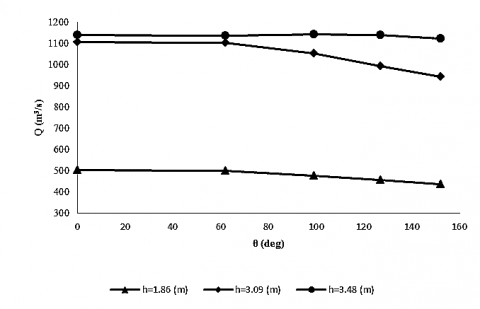Figure 13. The effect of the sector angle in the discharge flow rate of the morning glory spillway

In Figure 13, small drop in the discharge rate at the level of 505.48 meters (h=3.448 m) has occurred due to the fact the spillway is submerged at this elevation levels of the lake water and reached the maximum discharge capacity. Thus, the change in the geometry of the spillway crest will not have much effect on the discharge rate. Hence, one can say that the overflow in the pipe control mode does not follow the pattern of behavior in the crest control and orifice control modes in terms of the hydraulic behavior.

Changing the geometry of the spillway crest inlet opening changes the behavior of the water while overflowing from the crest edge. Due to the created sectors, some turbulence occurs when water enters the vertical shaft. This phenomenon occurs as the water hits the edges of the sector when overflowing the edges of the spillway and causes turmoil in the flow.

One can say that the created turbulence is the explaining factor for reducing the discharge rate observed in Figure 13. In addition, reducing the length of the watershed in the spillway crest caused by the creation of the sectors reduces the spillway discharge rate due, since according to Eq. (4), the discharge rate of the spillways is directly proportional to parameters C0 (spillway discharge coefficient), L (spillway length) and H0 (water heights on the crown). Since the creation of the sector reduces the length of the spillway, one can conclude that the decrease in length has reduced the amount of discharge.

$Q=C_{0} L H_{0}^{\frac{3}{2}}$    (4)

7.1.2 Investigating the effect of effective length reduction on the capacity of the spillway discharge

In the morning glory spillways without a sector, the effective length is equal to the circumference of the circle that covers the surrounding of the spillway (in this research, Le = 106.09 m). Obviously, by creating the sector and cutting the geometry of the morning glory spillway from the hypotenuses shown in Figure 10, the effective length of the catchment will be reduced. In Table 6, the values of discharge obtained from the software for the spillway without a sector for three water levels of the dam reservoir (respectively, the water levels related to the crest control, orifice control and pipe control) in simulation are given with the codes R1, R2 and R3 respectively as 503.36 (m3/s), 1104.44 (m3/s) and 1139.76 (m3/s).

In Table 6, the effective length reduction rate of the sectoral morning glory spillway is examined compared to a non-sectoral spillway by increased sector angle. Also, the effect of reducing effective length on the spillway discharge capacity is shown.

Due to the effective length reduction values, it can be demonstrated that in the modes of crest control and orifice control, there is a meaningful relationship between effective length reduction and reduction in the discharge capacity of the spillway. It should be noted that such a relationship is not found in the pipe control mode and the amount of spillway discharge capacity is independent of the effective length in this case.

Table 6. Estimation of the variation rate in the capacity of discharge of the sectoral morning glory spillway by increasing the sector angle (reducing the effective length of the spillway)

 Run No. Angle of Sector (θ) Reduction percentage of effective length h (m) Elv (m) Percentage of decrease in the flow rate R1 0° _ 1.86 503.86 _ R2 0° 3.09 505.09 R3 0° 3.48 505.48 R4 62° 0.0072 1.86 503.86 0.0027 R5 62° 3.09 505.09 0.0026 R6 62° 3.48 505.48 0.0031 R7 99° 0.0336 1.86 503.86 0.0499 R8 99° 3.09 505.09 0.0462 R9 99° 3.48 505.48 -0.0019 R10 127° 0.0683 1.86 503.86 0.0945 R11 127° 3.09 505.09 0.1006 R12 127° 3.48 505.48 0.0017 R13 152° 0.1137 1.86 503.86 0.1279 R14 152° 3.09 505.09 0.1471 R15 152° 3.48 505.48 0.0144

7.2 Investigating the effects of considering radial sections on the velocity vectors

## 14-.png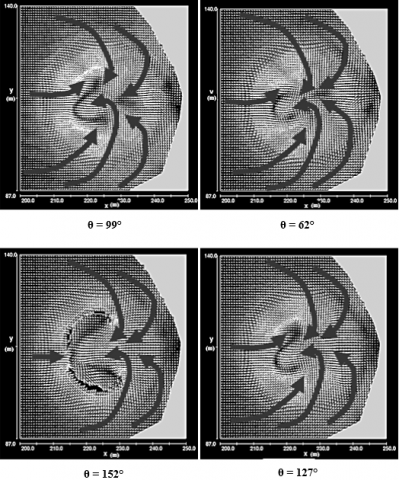Figure 14. Velocity vectors in the sectoral morning glory spillway; θ=62°, θ=99°, θ=127° and θ=152° in conditions the lake water is at a height of 505.48 m (pipe control)

The velocity vectors show the direction of flow movement in different points of the water. Based on these vectors, the direction of the water flow movement can be seen at different depths. In Figure 14, the velocity vector at the water surface is shown when entering the spillway to determine the formation of water flow vortices at the time of entering the morning glory spillway.

As seen in the Figure, the inflow from spillway upstream has a tangent velocity when entering the vertical shaft due to topographic conditions around the spillway. Thus, a rotary mode is created for the flow of water inside the vertical shaft and the water does not flow directly into the spillway.

As the angle of the sector increases, due to the loss of curvature inside the shaft at the site of the sector and creating corners inside the spillway, the rotation of the water flow is prevented to some point and the tangential velocity decreases. Finally, the water flow path will be shorter for discharge.

As the angle of the sector increases, the flow vortices gradually decrease and the depth of submergence reduces so that at the sectoral spillway (θ=152°), the flow submergence occurs within the shaft and lower than the spillway crest levels.

7.3 Regression analysis to find discharge rate equation

Investigating the flow of water in the non-sectoral morning glory spillway along with the sectors created in this research, one can show that, according to the nature of the problem, the discharge flow rate of the morning glory spillway is a function of the angle of the sector, the discharge coefficient and the height of the water passing over the spillway as seen in Eq. (5).

$Q=f\left(c_{d}, \theta, H_{0}\right)$    (5)

By extracting the regression model according to Eq. (6), we can show:

$Q=c_{d} K_{\theta} K_{H_{0}}$    (6)

To calculate the Kθ, it is worth mentioning that the effective length of the spillway affects the discharge of the morning glory spillway; thus, considering the θ as the angle of the sector, the effective length is calculated according to the relation (7):

$L_{e}=L_{1}+L_{2}$    (7)

and,

$\sin \left(\frac{\theta}{2}\right)=\frac{\left(L_{1} / 2\right)}{R} \Rightarrow L_{1}=2 \sin \left(\frac{\theta}{2}\right) R \quad L_{2}=(2 \pi-\theta) R$

So,

$\Rightarrow L_{e}=2 \sin \left(\frac{\theta}{2}\right) R+(2 \pi-\theta) R$    (8)

Regarding Eq. (7) and (8), we can say:

$K_{\theta}=\left(2 \sin \left(\frac{\theta}{2}\right) R+(2 \pi-\theta) R\right)^{a}$    (9)

And also:

$K_{H_{0}}=\left(H_{0}\right)^{b}$    (10)

Finally, according to relations (7) to (10), one can show that the discharge flow rate in the morning glory spillways is obtained by relation (11):

$Q=c_{d}\left(2 \sin \left(\frac{\theta}{2}\right) R+(2 \pi-\theta) R\right)^{a}\left(H_{0}\right)^{b}$    (11)

With the help of the least squares of errors, the exponents a and b as well as the coefficient of cd are extracted as follows:

$c_{d}=3.52 \quad a=0.88 \quad b=1.39$

Finally, the equation proposed for calculating the discharge flow rate in the morning glory spillways will be as follows:

$Q=3.52\left(2 \sin \left(\frac{\theta}{2}\right) R+(2 \pi-\theta) R\right)^{0.88}\left(H_{0}\right)^{1.39}$    (12)

The statistical error measurement parameters can be used to more accurately examine the measurement error. To this end, the data correlation rate (R2) has been investigated in Figure 15.

## 15-.png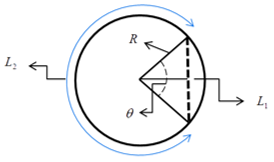Figure 15. A schematic view of the morning glory spillway plan to calculate the effective length

## 16.png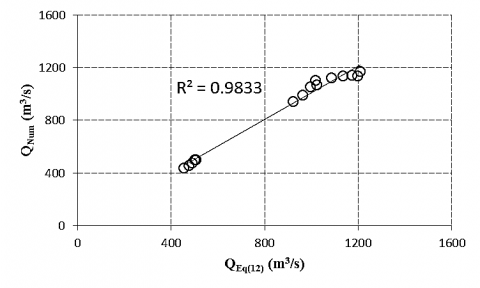Figure 16. Display of correlation of discharge flow rate obtained from the numerical model and the Eq. (12)

The results of the correlation diagram (R2) between the discharge rate obtained from Eq. (12) and the numerical model shown in Figure 16 indicate that an acceptable correlation is established between the outputs of the numerical model and the Eq. (12). The parameters of MAE (mean absolute error) and RMSE (Root Mean Square Error) obtained also confirm the conformity of the results obtained from Eq. (12) (Table (7)).

In Table (8), the error rate of the sectoral morning glory spillway has been investigated at different sectors angles separately. The maximum error rate is 7.77 % that has occurred at the elevation of 505.09 m (h=3.09 m) and in the spillway with a 62 degrees sector.

Table 7. Statistical parameters of error evaluation

 θ  = 0° θ  = 62° θ  = 99° θ  = 127° θ  = 152° RMSE 0.34 0.61 0.39 0.21 0.26 MAE 0.29 0.49 0.34 0.18 0.25

Table 8. Review of hydraulic properties and evaluating the error of sectoral morning glory spillway modeled in the software and obtained from the Eq. (12)

 Run No. Angle of Sector (θ) Elv (m) h (m) Q Num (m3/s) Q Eq (m3/s) Err (%) 1 _ 503.86 1.86 501.22 505.42 -0.84 2 _ 505.09 3.09 1070.00 1023.46 4.35 3 _ 505.48 3.48 1172.37 1207.32 -2.98 4 62° 503.86 1.86 502.02 501.73 0.06 5 62° 505.09 3.09 1101.61 1016.00 7.77 6 62° 505.48 3.48 1136.25 1198.52 -5.48 7 99° 503.86 1.86 478.24 490.73 -2.61 8 99° 505.09 3.09 1053.42 993.72 5.67 9 99° 505.48 3.48 1141.95 1172.24 -2.65 10 127° 503.86 1.86 455.77 475.09 -4.24 11 127° 505.09 3.09 993.30 962.04 3.15 12 127° 505.48 3.48 1137.78 1134.87 0.26 13 152° 503.86 1.86 438.96 454.64 -3.57 14 152° 505.09 3.09 941.98 920.63 2.27 15 152° 505.48 3.48 1123.30 1086.02 3.32

In Table (8), Elv is elevation of the fluid free surface, h is height of water on the spillway, QNum is discharge rate obtained from the numerical model, QEq is discharge rate obtained from the Eq. (12), Err is error rate obtained from Eq. (3).

8. Conclusions

In this study, we tried to model the flow by a three-dimensional approach around the sectoral morning glory spillway using the Flow-3D numerical model.

Using the results extracted from the numerical model, the curve charts of the spillway performance were compared with the experimental results and the simulation error rate was determined.

Then, by changing the spillway geometry and creating sections with four different angles on the crest, the discharge flow rate of the spillway was calculated with the help of the software by considering the three different water elevation for the dam reservoir.

Finally, by extracting the correlation regression model, a relation was provided for the discharge rate of the sectoral morning glory spillway. Comparing the results obtained from the proposed relation and the numerical model, it was found that with the help of the relation, the discharge rate of the sectoral morning glory spillway can be calculated with a small error rate (maximum error equal to 7.77 %).

In sectoral spillways, in the case where the height of the water from the edge of the spillway crest is considered constant, the discharge rate decreases as the angle of the sector (θ) increase. Thus, at the sectoral morning glory spillway of θ=152°, at the highest mode (H0=1.86m), we see a 12.24 % decrease in the discharge rate compared to the non-sectoral spillway.

In the sectoral spillways, in the case where the sector angle (θ) is considered constant, the discharge rate increases as the water height from the edge of the spillway crest increases. Thus, in the sectoral spillway, with increasing water height from H0=1.86m to H0=3.48 m, 134 % increase occurs in the discharge rate, while in the sectoral spillway of θ=152°, with the same increase in water height, we see a 160 % increase in the discharge rate. This difference, reflects the fact that by creating a sector in the morning glory spillway, we witness a wider range of discharge rates. In the sectoral spillways, with the rise in the elevation of the dam reservoir water, the distance between the minimum and maximum discharge rates is higher than the non-sectoral morning glory spillway.

Nomenclature
 u, v, w velocities in the x-, y-, and z-directions, m.s-1 VF fluid volume fraction Ax, Ay, Az ρ fractional areas, m2 density, kg.m-3 ρ' pressure, N.m-2 gi ravity force, m.s-2 fi Reynolds stresses Elv reservoir water elevation, m h spillway crest distance from the free flow surface, m Q flow (discharge) rates, m3.s-1 Le effective length of morning glory spillway, m Greek symbols Ɵ Angle sectors, degree
References

 Fattor, C.A., Bacchiega J.D. (2009). Design conditions for morning-glory spillways: Application to Potrerillos dam spillway. Advances in Water Resources and Hydraulic Engineering, Springer, Berlin, Heidelberg. https://doi.org/10.1007/978-3-540-89465-0_364

 Savic, L., Kapor, R., Kuzmanovic, V., Milovanovic, B. (2014). Shaft spillway with a deflector downstream of the vertical bend. Proceedings of the Institution of Civil Engineers - Water Management, 167(5): 269-278. https://doi.org/10.1680/wama.12.00111

 Nohani, E. (2015). An experimental study on the effect of vortex breakers thickness on discharge efficiency for shaft spillways. Science International-Lahore, 2295-2299

 Fais, L.M.C.F., Filho, J.G.D., Genovez, A.I.B. (2015). Geometry influence and discharge curve correction in morning glory spillways. Proceedings of the 36th IAHR World Congress.

 Zhang, X.Q. (2015). Hydraulic characteristics of the rotational flow shaft spillway for high dams. International Journal of Heat and Technology, 33(1): 167-174. https://doi.org/10.18280/ijht.330123

 Alfatlawi, T.J., Alshakli, H.I (2015). Prediction the coefficient of discharge for stepped morning glory spillway using ANN and MNLR approaches. International Journal of Civil and Environmental Engineering, 37(2): 1701-8285.

 Nohani, E. (2015). Numerical simulation of the flow pattern on morning glory spillways. International Journal of Life Sciences, 9(4): 28-31. https://doi.org/10.3126/ijls.v9i4.12671

 Shemshi, R., Kabiri-Samani, A. (2016). Swirling flow at vertical shaft spillways with circular piano-key inlets. Journal of Hydraulic Research, 55(2): 248-258. https://doi.org/10.1080/00221686.2016.1238015

 Musavi-Jahromi, S.H., Hajipour, G., Eghdam, M. (2016). Discharge coefficient in the morning glory spillways due to longitudinal angles of vortex breakers. Bulletin of environment. Pharmacology and Life Sciences, 5(5): 34-41.

 http://gilnegah.ir, accessed on Jan. 17, 2019.

 http://gostareshtolidtejaratgilan.ir, accessed on Jan. 17, 2019.

 Khorasani-Zadeh, A. (2015). Hydraulic modeling studies of the morning glory spillway of Haraz dam, Report on determining the spillway discharge capacity. Water Research Institute Iran.

 http://daminfo.wrm.ir/fa/pageviewreport/761, accessed on March 17, 2019.